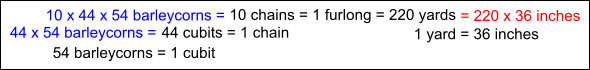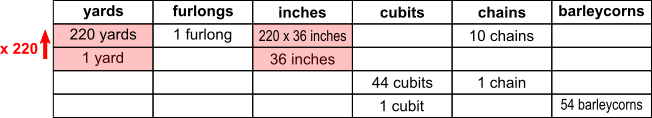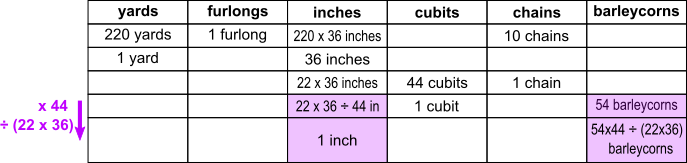#### You may also like### Building Tetrahedra

Can you make a tetrahedron whose faces all have the same perimeter?A 1 metre cube has one face on the ground and one face against a wall. A 4 metre ladder leans against the wall and just touches the cube. How high is the top of the ladder above the ground?Four rods are hinged at their ends to form a convex quadrilateral. Investigate the different shapes that the quadrilateral can take. Be patient this problem may be slow to load.

# Inches and Barleycorns

##### Age 14 to 16 Short Challenge Level:

Both of the methods shown use diagrams to organise the information given. There are also good methods which do not use diagrams, but involve similar calculations.

Working out how many inches and how many barleycorns there are in a furlong$220\times36$ inches = $10\times44\times54$ barleycorns

$1$ inch = $\dfrac{10\times44\times54}{220\times36}=\dfrac{10\times(11\times4)\times(9\times6)}{(11\times2\times10)\times(9\times4)}=3$ barleycorns

Working in inches using a table$(54\times44)\div(22\times36) = (6\times9\times4\times11) \div (2\times11\times6\times 6)=3$

You can find more short problems, arranged by curriculum topic, in our short problems collection.